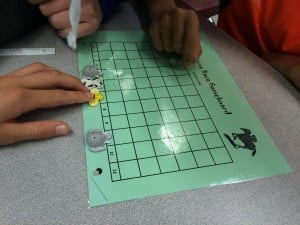May 24

# What’s the Chance?Probability is chance or how likely something will happen.  Having a good grasp of fractions, decimals, and percents certainly helps with understanding probability because probability is often expressed as a fraction or decimal or percent. For example, we often hear the chance of rain expressed as a percent such as a 60 % chance or 0% chance.

We’ve been conducting some experiments using spinners and plotting our results on a lineplot. We’ve learned there is a difference between theoretical probability (what we expect to see happen) and experimental probability (what actually happened).

We can show the likeliness that an event can occur by using a probability line where 0 is impossible and 1 is certain.Of course, you can’t have a probability unit without some engaging and interactive games! So far, we’ve played SKUNK, PIG, and recently a horse race game.

Have a look!

Click on the links below to play some probability games and practice your skills!

Study Jams

Kids Math Games

Probability Games

What is your favourite probability game?

The following two tabs change content below.